# Faces dataset decompositions¶

This example applies to The Olivetti faces dataset different unsupervised matrix decomposition (dimension reduction) methods from the module sklearn.decomposition (see the documentation chapter Decomposing signals in components (matrix factorization problems)).

• Authors: Vlad Niculae, Alexandre Gramfort

## Dataset preparation¶

import logging

from numpy.random import RandomState
import matplotlib.pyplot as plt

from sklearn.datasets import fetch_olivetti_faces
from sklearn import cluster
from sklearn import decomposition

rng = RandomState(0)

# Display progress logs on stdout
logging.basicConfig(level=logging.INFO, format="%(asctime)s %(levelname)s %(message)s")

faces, _ = fetch_olivetti_faces(return_X_y=True, shuffle=True, random_state=rng)
n_samples, n_features = faces.shape

# Global centering (focus on one feature, centering all samples)
faces_centered = faces - faces.mean(axis=0)

# Local centering (focus on one sample, centering all features)
faces_centered -= faces_centered.mean(axis=1).reshape(n_samples, -1)

print("Dataset consists of %d faces" % n_samples)

Dataset consists of 400 faces


Define a base function to plot the gallery of faces.

n_row, n_col = 2, 3
n_components = n_row * n_col
image_shape = (64, 64)

def plot_gallery(title, images, n_col=n_col, n_row=n_row, cmap=plt.cm.gray):
fig, axs = plt.subplots(
nrows=n_row,
ncols=n_col,
figsize=(2.0 * n_col, 2.3 * n_row),
facecolor="white",
constrained_layout=True,
)
fig.set_edgecolor("black")
fig.suptitle(title, size=16)
for ax, vec in zip(axs.flat, images):
vmax = max(vec.max(), -vec.min())
im = ax.imshow(
vec.reshape(image_shape),
cmap=cmap,
interpolation="nearest",
vmin=-vmax,
vmax=vmax,
)
ax.axis("off")

fig.colorbar(im, ax=axs, orientation="horizontal", shrink=0.99, aspect=40, pad=0.01)
plt.show()


Let’s take a look at our data. Gray color indicates negative values, white indicates positive values.

plot_gallery("Faces from dataset", faces_centered[:n_components])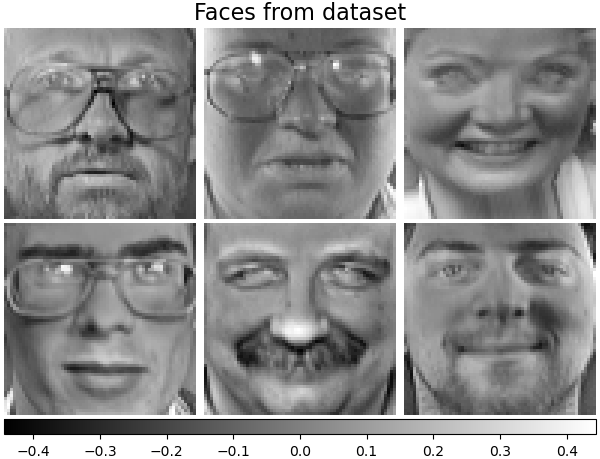## Decomposition¶

Initialise different estimators for decomposition and fit each of them on all images and plot some results. Each estimator extracts 6 components as vectors $$h \in \mathbb{R}^{4096}$$. We just displayed these vectors in human-friendly visualisation as 64x64 pixel images.

Read more in the User Guide.

### Eigenfaces - PCA using randomized SVD¶

Linear dimensionality reduction using Singular Value Decomposition (SVD) of the data to project it to a lower dimensional space.

Note

The Eigenfaces estimator, via the sklearn.decomposition.PCA, also provides a scalar noise_variance_ (the mean of pixelwise variance) that cannot be displayed as an image.

pca_estimator = decomposition.PCA(
n_components=n_components, svd_solver="randomized", whiten=True
)
pca_estimator.fit(faces_centered)
plot_gallery(
"Eigenfaces - PCA using randomized SVD", pca_estimator.components_[:n_components]
)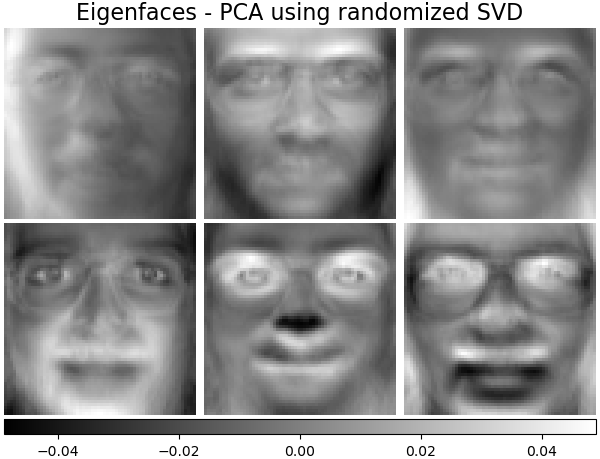### Non-negative components - NMF¶

Estimate non-negative original data as production of two non-negative matrices.

nmf_estimator = decomposition.NMF(n_components=n_components, tol=5e-3)
nmf_estimator.fit(faces)  # original non- negative dataset
plot_gallery("Non-negative components - NMF", nmf_estimator.components_[:n_components])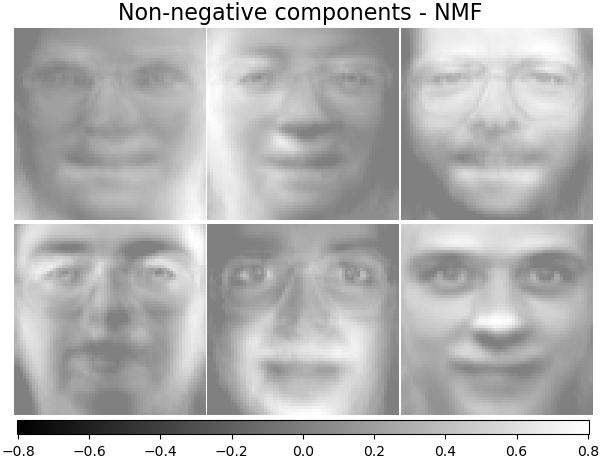### Independent components - FastICA¶

Independent component analysis separates a multivariate vectors into additive subcomponents that are maximally independent.

ica_estimator = decomposition.FastICA(
n_components=n_components, max_iter=400, whiten="arbitrary-variance", tol=15e-5
)
ica_estimator.fit(faces_centered)
plot_gallery(
"Independent components - FastICA", ica_estimator.components_[:n_components]
)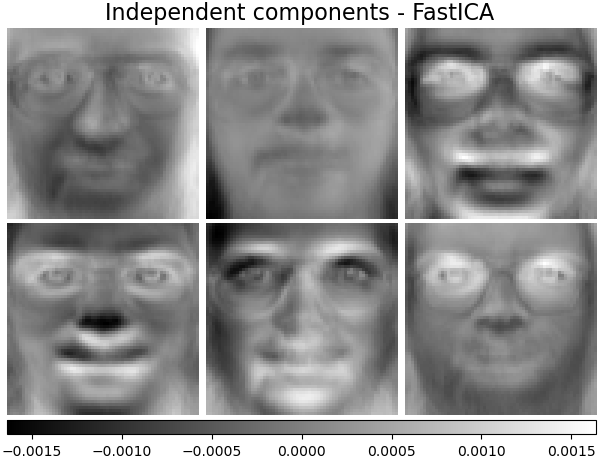### Sparse components - MiniBatchSparsePCA¶

Mini-batch sparse PCA (MiniBatchSparsePCA) extracts the set of sparse components that best reconstruct the data. This variant is faster but less accurate than the similar sklearn.decomposition.SparsePCA.

batch_pca_estimator = decomposition.MiniBatchSparsePCA(
n_components=n_components, alpha=0.1, max_iter=100, batch_size=3, random_state=rng
)
batch_pca_estimator.fit(faces_centered)
plot_gallery(
"Sparse components - MiniBatchSparsePCA",
batch_pca_estimator.components_[:n_components],
)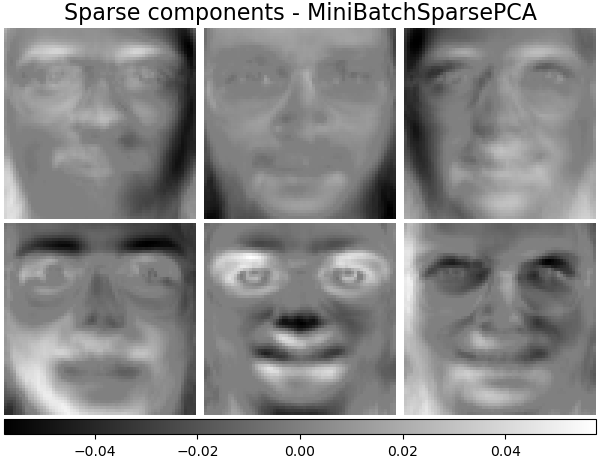### Dictionary learning¶

By default, MiniBatchDictionaryLearning divides the data into mini-batches and optimizes in an online manner by cycling over the mini-batches for the specified number of iterations.

batch_dict_estimator = decomposition.MiniBatchDictionaryLearning(
n_components=n_components, alpha=0.1, max_iter=50, batch_size=3, random_state=rng
)
batch_dict_estimator.fit(faces_centered)
plot_gallery("Dictionary learning", batch_dict_estimator.components_[:n_components])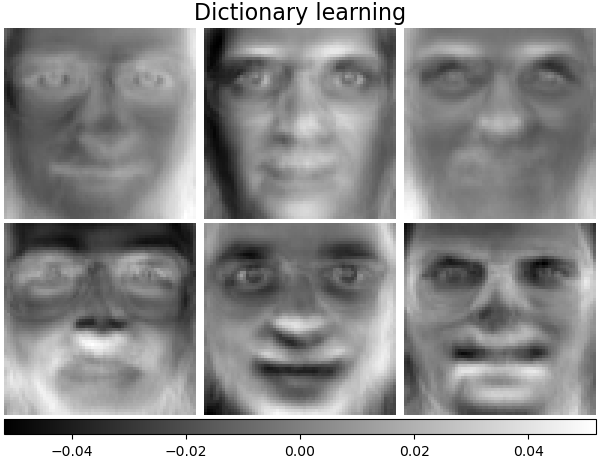### Cluster centers - MiniBatchKMeans¶

MiniBatchKMeans is computationally efficient and implements on-line learning with a partial_fit method. That is why it could be beneficial to enhance some time-consuming algorithms with MiniBatchKMeans.

kmeans_estimator = cluster.MiniBatchKMeans(
n_clusters=n_components,
tol=1e-3,
batch_size=20,
max_iter=50,
random_state=rng,
n_init="auto",
)
kmeans_estimator.fit(faces_centered)
plot_gallery(
"Cluster centers - MiniBatchKMeans",
kmeans_estimator.cluster_centers_[:n_components],
)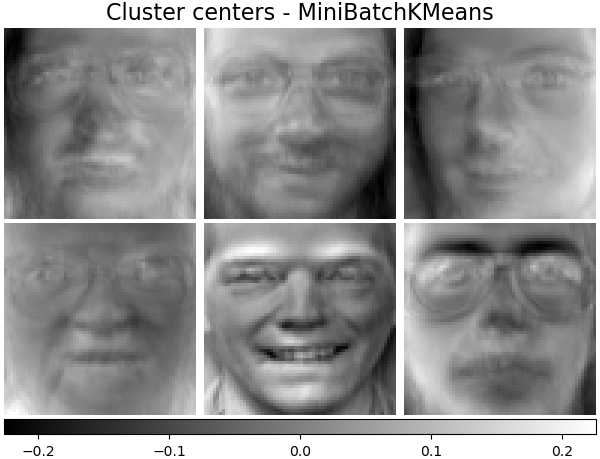### Factor Analysis components - FA¶

Factor Analysis is similar to PCA but has the advantage of modelling the variance in every direction of the input space independently (heteroscedastic noise). Read more in the User Guide.

fa_estimator = decomposition.FactorAnalysis(n_components=n_components, max_iter=20)
fa_estimator.fit(faces_centered)
plot_gallery("Factor Analysis (FA)", fa_estimator.components_[:n_components])

# --- Pixelwise variance
plt.figure(figsize=(3.2, 3.6), facecolor="white", tight_layout=True)
vec = fa_estimator.noise_variance_
vmax = max(vec.max(), -vec.min())
plt.imshow(
vec.reshape(image_shape),
cmap=plt.cm.gray,
interpolation="nearest",
vmin=-vmax,
vmax=vmax,
)
plt.axis("off")
plt.title("Pixelwise variance from \n Factor Analysis (FA)", size=16, wrap=True)
plt.show()

•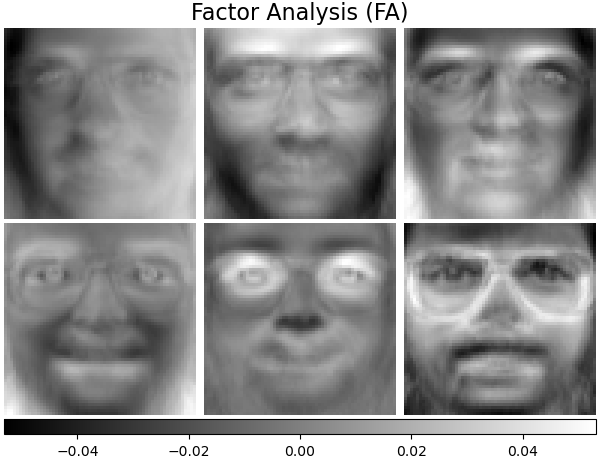•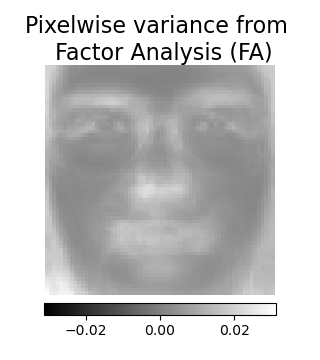## Decomposition: Dictionary learning¶

In the further section, let’s consider Dictionary Learning more precisely. Dictionary learning is a problem that amounts to finding a sparse representation of the input data as a combination of simple elements. These simple elements form a dictionary. It is possible to constrain the dictionary and/or coding coefficients to be positive to match constraints that may be present in the data.

MiniBatchDictionaryLearning implements a faster, but less accurate version of the dictionary learning algorithm that is better suited for large datasets. Read more in the User Guide.

Plot the same samples from our dataset but with another colormap. Red indicates negative values, blue indicates positive values, and white represents zeros.

plot_gallery("Faces from dataset", faces_centered[:n_components], cmap=plt.cm.RdBu)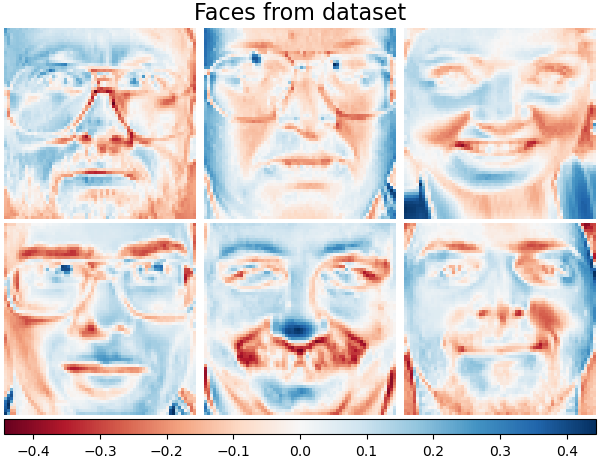Similar to the previous examples, we change parameters and train MiniBatchDictionaryLearning estimator on all images. Generally, the dictionary learning and sparse encoding decompose input data into the dictionary and the coding coefficients matrices. $$X \approx UV$$, where $$X = [x_1, . . . , x_n]$$, $$X \in \mathbb{R}^{m×n}$$, dictionary $$U \in \mathbb{R}^{m×k}$$, coding coefficients $$V \in \mathbb{R}^{k×n}$$.

Also below are the results when the dictionary and coding coefficients are positively constrained.

### Dictionary learning - positive dictionary¶

In the following section we enforce positivity when finding the dictionary.

dict_pos_dict_estimator = decomposition.MiniBatchDictionaryLearning(
n_components=n_components,
alpha=0.1,
max_iter=50,
batch_size=3,
random_state=rng,
positive_dict=True,
)
dict_pos_dict_estimator.fit(faces_centered)
plot_gallery(
"Dictionary learning - positive dictionary",
dict_pos_dict_estimator.components_[:n_components],
cmap=plt.cm.RdBu,
)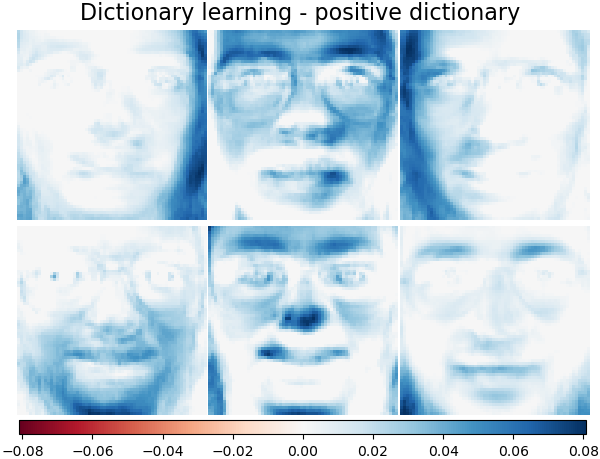### Dictionary learning - positive code¶

Below we constrain the coding coefficients as a positive matrix.

dict_pos_code_estimator = decomposition.MiniBatchDictionaryLearning(
n_components=n_components,
alpha=0.1,
max_iter=50,
batch_size=3,
fit_algorithm="cd",
random_state=rng,
positive_code=True,
)
dict_pos_code_estimator.fit(faces_centered)
plot_gallery(
"Dictionary learning - positive code",
dict_pos_code_estimator.components_[:n_components],
cmap=plt.cm.RdBu,
)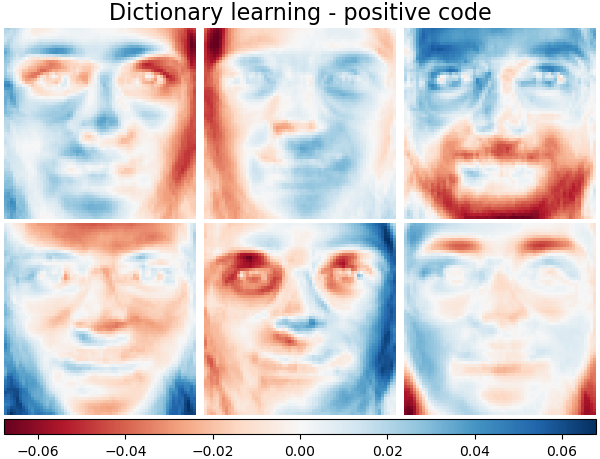### Dictionary learning - positive dictionary & code¶

Also below are the results if the dictionary values and coding coefficients are positively constrained.

dict_pos_estimator = decomposition.MiniBatchDictionaryLearning(
n_components=n_components,
alpha=0.1,
max_iter=50,
batch_size=3,
fit_algorithm="cd",
random_state=rng,
positive_dict=True,
positive_code=True,
)
dict_pos_estimator.fit(faces_centered)
plot_gallery(
"Dictionary learning - positive dictionary & code",
dict_pos_estimator.components_[:n_components],
cmap=plt.cm.RdBu,
)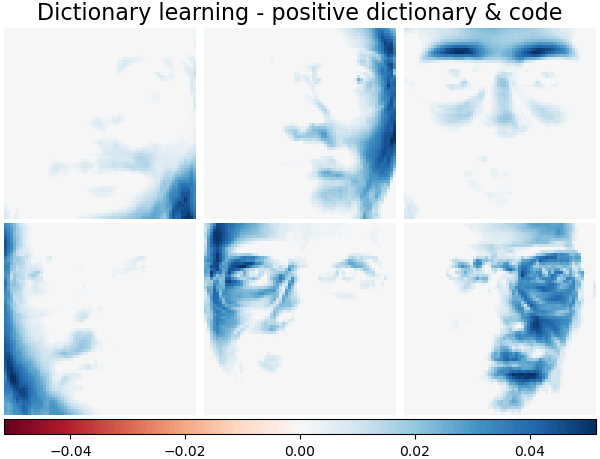Total running time of the script: ( 0 minutes 10.198 seconds)

Gallery generated by Sphinx-Gallery